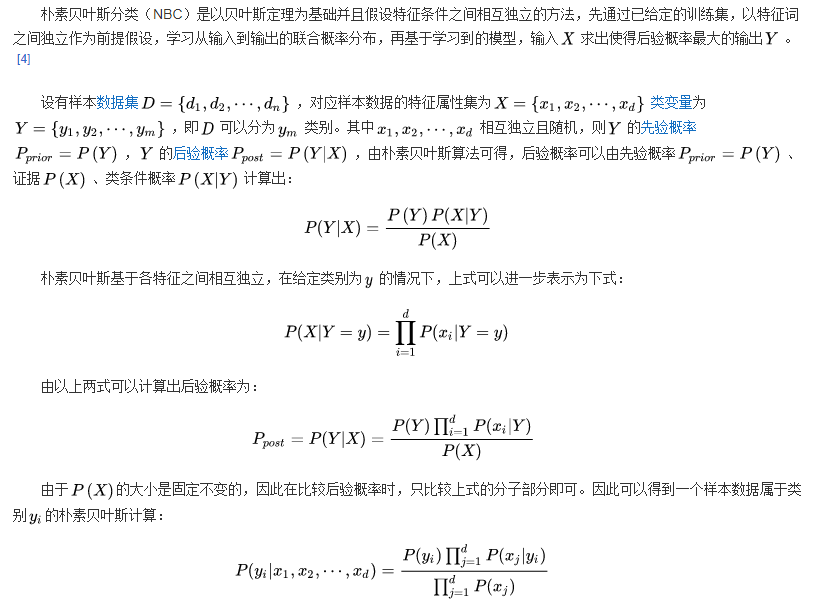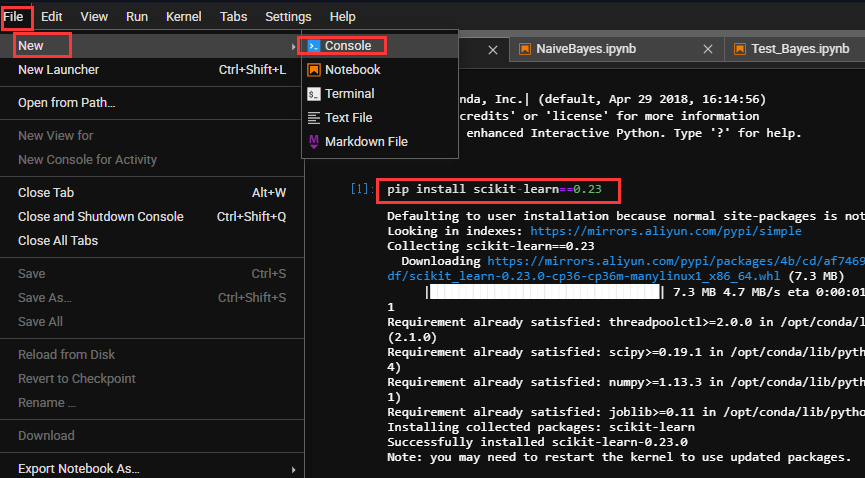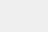• 常规操作： import numpy as np import ...min, x2_max) plt.title(u'GaussianNB对鸢尾花数据的分类结果, 正确率:%.3f%%' % (100 * accuracy_score(y_test, y_test_hat)), fontsize=18) plt.grid(True) plt.show()
常规操作：import numpy as npimport pandas as pdimport matplotlib.pyplot as pltimport matplotlib as mplfrom sklearn.preprocessing import StandardScaler, MinMaxScaler, PolynomialFeaturesfrom sklearn.naive_bayes import GaussianNB, MultinomialNB#高斯贝叶斯和多项式朴素贝叶斯from sklearn.pipeline import Pipelinefrom sklearn.metrics import accuracy_scorefrom sklearn.model_selection import train_test_splitfrom sklearn.neighbors import KNeighborsClassifier## 设置属性防止中文乱码mpl.rcParams['font.sans-serif'] = [u'SimHei']mpl.rcParams['axes.unicode_minus'] = False# 花萼长度、花萼宽度，花瓣长度，花瓣宽度iris_feature_E = 'sepal length', 'sepal width', 'petal length', 'petal width'iris_feature_C = u'花萼长度', u'花萼宽度', u'花瓣长度', u'花瓣宽度'iris_class = 'Iris-setosa', 'Iris-versicolor', 'Iris-virginica'features = [2,3]## 读取数据path = './datas/iris.data'  # 数据文件路径data = pd.read_csv(path, header=None)x = data[list(range(4))]x = x[features]y = pd.Categorical(data).codes ## 直接将数据特征转换为0，1,2print ("总样本数目：%d；特征属性数目：%d" % x.shape)总样本数目：150；特征属性数目：2数据分割，形成模型训练数据和测试数据x_train1, x_test1, y_train1, y_test1 = train_test_split(x, y, train_size=0.8, random_state=14)x_train, x_test, y_train, y_test = x_train1, x_test1, y_train1, y_test1print ("训练数据集样本数目：%d, 测试数据集样本数目：%d" % (x_train.shape, x_test.shape))训练数据集样本数目：120, 测试数据集样本数目：30高斯贝叶斯模型构建clf = Pipeline([('sc', StandardScaler()),#标准化，把它转化成了高斯分布('poly', PolynomialFeatures(degree=1)),('clf', GaussianNB())]) # MultinomialNB多项式贝叶斯算法中要求特征属性的取值不能为负数## 训练模型clf.fit(x_train, y_train)Pipeline(memory=None,steps=[('sc', StandardScaler(copy=True, with_mean=True, with_std=True)), ('poly', PolynomialFeatures(degree=1, include_bias=True, interaction_only=False)), ('clf', GaussianNB(priors=None))])计算预测值并计算准确率y_train_hat = clf.predict(x_train)print ('训练集准确度: %.2f%%' % (100 * accuracy_score(y_train, y_train_hat)))y_test_hat = clf.predict(x_test)print ('测试集准确度：%.2f%%' % (100 * accuracy_score(y_test, y_test_hat)))训练集准确度: 95.83%测试集准确度：96.67%产生区域图N, M = 500, 500     # 横纵各采样多少个值x1_min1, x2_min1 = x_train.min()x1_max1, x2_max1 = x_train.max()x1_min2, x2_min2 = x_test.min()x1_max2, x2_max2 = x_test.max()x1_min = np.min((x1_min1, x1_min2))x1_max = np.max((x1_max1, x1_max2))x2_min = np.min((x2_min1, x2_min2))x2_max = np.max((x2_max1, x2_max2))t1 = np.linspace(x1_min, x1_max, N)t2 = np.linspace(x2_min, x2_max, N)x1, x2 = np.meshgrid(t1, t2)  # 生成网格采样点x_show = np.dstack((x1.flat, x2.flat)) # 测试点cm_light = mpl.colors.ListedColormap(['#77E0A0', '#FF8080', '#A0A0FF'])cm_dark = mpl.colors.ListedColormap(['g', 'r', 'b'])y_show_hat = clf.predict(x_show)      # 预测值y_show_hat = y_show_hat.reshape(x1.shape)画图plt.figure(facecolor='w')plt.pcolormesh(x1, x2, y_show_hat, cmap=cm_light)     # 预测值的显示plt.scatter(x_train[features], x_train[features], c=y_train, edgecolors='k', s=50, cmap=cm_dark)plt.scatter(x_test[features], x_test[features], c=y_test, marker='^', edgecolors='k', s=120, cmap=cm_dark)plt.xlabel(iris_feature_C[features], fontsize=13)plt.ylabel(iris_feature_C[features], fontsize=13)plt.xlim(x1_min, x1_max)plt.ylim(x2_min, x2_max)plt.title(u'GaussianNB对鸢尾花数据的分类结果, 正确率:%.3f%%' % (100 * accuracy_score(y_test, y_test_hat)), fontsize=18)plt.grid(True)plt.show()展开全文• Task05 基于鸢尾花数据集的贝叶斯分类算法实践 一、学习内容概括 学习资料： 1.阿里云天池-AI训练营机器学习：https://tianchi.aliyun.com/specials/promotion/aicampml?invite_channel=1&accounttraceid=7df...
Task05 基于鸢尾花数据集的贝叶斯分类算法实践

一、学习内容概括

学习资料：

1.阿里云天池-AI训练营机器学习：https://tianchi.aliyun.com/specials/promotion/aicampml?invite_channel=1&accounttraceid=7df048c2ce194081b514fd2c8e9a3f00cqmm

2.sklearnAPI：https://scikit-learn.org/stable/modules/classes.html

二、具体学习内容

朴素贝叶斯算法原理：由上述原理，分析出朴素贝叶斯解决什么问题：

样本数据集D和对应的特征属性集X可以合二为一形成一个二维数组（样本数n_samples，特征数n_features），我们把这个二维数组称为数据集。原理中提到的类变量Y，我们也形成一个一维数组（样本数n_samples，），我们把这个一维数组称为标签集。从整个数据集中可以再划分出训练集和测试集，标签集也相应划分出训练标签集和测试标签集。

我们先输入训练集和对应的标签集进行学习，通过学习得到朴素贝叶斯模型。基于模型，输入某个样本X，就可以得到相应的标签预测概率值集P(Y|X)（X条件下Y的概率），这里为什么说是一个值集，因为Y中有很多类别，针对一个样本，属于什么类别，每种类别都有其预测概率值P(Yi|X)，有的值大有的值小，其实这个值集在代码中表示就是一个（1，标签数）型的二维数组（当然这是针对一个样本而言，多个样本就是（样本数，标签数））。通过预测结果，我们可以直接看出P(Y|X)中最大的那个概率值，这个概率值对应的类别就是我们想知道的类别Yi。这就是朴素贝叶斯解决的问题。另外，我们把P(Y|X)记为Y的后验概率。

说到这里还是比较抽象，下面从代码中一步步解读。

代码流程：

Step1: 库函数导入
Step2: 数据导入&分析
Step3: 模型训练
Step4: 模型预测
1 库函数导入

import warnings
warnings.filterwarnings('ignore')
import numpy as np
# 加载莺尾花数据集
from sklearn import datasets
# 导入高斯朴素贝叶斯分类器
from sklearn.naive_bayes import GaussianNB
from sklearn.model_selection import train_test_split

1.1 忽略警告信息

Q：在python中，代码可以正常运行但是会提示警告。

A：调用warnings模块中警告过滤器filterwarnings()函数忽略警告消息。

1.2 sklearn.naive_bayes：朴素贝叶斯。其中naive_bayes.GaussianNB（* [，先验，...]）：高斯朴素贝叶斯（GaussianNB）

https://scikit-learn.org/stable/modules/generated/sklearn.naive_bayes.GaussianNB.html#sklearn.naive_bayes.GaussianNB

1.3 sklearn.model_selection：选型。model_selection.train_test_split（*数组，...）用于数据集的分割，分割成训练集和测试集

https://scikit-learn.org/stable/modules/generated/sklearn.model_selection.train_test_split.html#sklearn.model_selection.train_test_split

2 数据导入&分析

## return_X_y默认为False，如果为True，表示返回的是（data，target）
## X=data是（样本数，特征数）数组；y=target是（1，标签数）数组
X, y = datasets.load_iris(return_X_y=True)
## X,y是待拆分的数据集；test_size是指将0.2比例的数据集拿出来作为测试集；
## random_state默认值为None,不设置则每次复现代码时都会随机分割，如果设置为一个值，那复现代码时会是与本次一样的分割结果
X_train, X_test, y_train, y_test = train_test_split(X, y, test_size=0.2, random_state=0)

这里可以看一下各个数据的形状：X(150,4)、y(150,)、X_train(120,4)、X_test(30,4)、y_train(120,)、y_test(30,4)。看得出来150*0.2=30。这里的X就是数据集，y就是标签集。X_train和X_test是从X中划分出的训练集、测试集；y_train、y_test是从y中划分出的与X相对应的标签集。

通过分析发现训练数据是数值类型的数据，这里假设每个特征服从高斯分布，因此我们选择高斯朴素贝叶斯来进行分类计算。高斯朴素贝叶斯假设每个特征都服从高斯分布，我们把一个随机变量X服从数学期望为μ，方差为σ^2的数据分布称为高斯分布。对于每个特征我们一般使用平均值来估计μ和使用所有特征的方差估计σ^2。

3 模型训练

# 使用高斯朴素贝叶斯进行计算
## var_smoothing：float，默认值= 1e-9。表示所有特征的最大方差部分，已添加到方差中以提高计算稳定性。
## var_smoothing是sklearn0.20版中的新功能
clf = GaussianNB(var_smoothing=1e-8)
clf.fit(X_train, y_train)

我们根据X_train和y_train训练出高斯朴素贝叶斯模型。

通过sklearn.__version__检查sklearn版本，发现是“0.19”，var_smoothing是sklearn0.20版中的新功能，所以var_smoothing不能用。因此我们在控制台更新sklearn版本：pip install scikit-learn==0.234 模型预测

# 评估
## GaussianNB.predict（X）对测试向量X进行分类，返回X的预测目标值。
## 输入(n_samples, n_features)型数组。输出(n_samples,)型数组。
## X_test.shape（30,4）,y_pred.shape(30,)
y_pred = clf.predict(X_test)
print(y_pred.shape,"个预测值:",y_pred)

## 测试集标签值y_test与估计值y_pred比较，共30个测试样本。得到一个正确率acc
acc = np.sum(y_test == y_pred) / X_test.shape
print("Test Acc : %.3f" % acc)

输出结果：

30 个预测值: [2 1 0 2 0 2 0 1 1 1 1 1 1 1 1 0 1 1 0 0 2 1 0 0 2 0 0 1 1 0]
Test Acc : 0.967

通过训练得到的模型，在测试集上表现不错，正确率达到96.7%。

基于训练好的模型，对X_test进行一个预测，与y_test对比来对模型进行一个评估。

# 预测
## GaussianNB.predict_proba（X）对测试向量X的概率估计。输入、输出都是(n_samples, n_features)型数组，
## X_test[:1]数组切片，只取一行样本
y_proba = clf.predict_proba(X_test[:1])

print("\n测试样本",X_test[:1],"的预测类别为：",clf.predict(X_test[:1]))
print("\n测试样本",X_test[:1],"的预测概率为：\n",y_proba)

输出结果：

测试样本 [[5.8 2.8 5.1 2.4]] 的预测类别为： 

测试样本 [[5.8 2.8 5.1 2.4]] 的预测概率为：
[[1.63542393e-232 2.18880483e-006 9.99997811e-001]]

输入一个样本，不光可以根据predict()函数直接得到其预测类别，还可以根据predict_proba()函数看到针对每一种类别/标签，预测的概率都是多少，几个预测概率值相比较，最大的那个预测值9.99997811e-001对应的类别就是我们想要的类别，也就是predict()函数直接预测到的那个。

重复一遍朴素贝叶斯的功能，就是输入样本，根据学习好的模型进行预测，预测出样本属于几种类别的概率，其中，几种类别预测概率值中最大的那个对应的类别就是我们想要的类别Yi。也就是说事件X最有可能的结果是Yi。

从上述例子中的预测结果中，我们可以看到类别2对应的后验概率值最大，所以我们认为类别2是最优的结果。

三、学习总结

sklearn对朴素贝叶斯算法进行了封装，我们就像在使用一个黑盒子。这个例子只是展示了贝叶斯算法的使用，并没有展现算法的具体细节。


展开全文机器学习
• 使用的贝叶斯分类算法实现的，编程语言为java。是我本学期修的数据库与数据挖掘的课程的期末课程作业，算法本身不难，思路理清楚了很简单。先看看鸢尾花(Iris)数据集(下图为数据集的部分截图)，鸢尾花有setosa、...
使用的贝叶斯分类算法实现的，编程语言为java。是我本学期修的数据库与数据挖掘的课程的期末课程作业，算法本身不难，思路理清楚了很简单。先看看鸢尾花(Iris)数据集(下图为数据集的部分截图)，鸢尾花有setosa、Versicolor、Virginica3个类别，数据集中各个类别各50条数据，一共是150条数据记录，每条数据记录的前4个值分别表示鸢尾花的sepalLength、sepalWidth、petalLengthpetalWidth，第5个值是鸢尾花的类型。算法实现过程中将每个类别的前40条记录作为训练数据，进行分类模型的训练，每个类别的后10条数据作为测试数据，对分类模型的准确性进行判断。算法基本思路：由概率论中先验概率与后验概率的转换公式可以得到：设C为鸢尾花类别，F1，F2，F3，F4表示鸢尾花的4个特征，由于可见，分母与类别没有关系，在分类时不提供判别信息，不作考虑。因此，分类只与分子有关，，又假设F1，F2，F3，F4是相互独立，所以分子等价于：由于都有P(C)项，所以可以就只计算即计算各特征分别属于各类别的概率，然后相乘，值最大的则是那个相应的类别。所以鸢尾花分类的思路为：先分别计算3个类别分别在4个特征上的均值和方差，然后构造相应的概率密度函数，在对测试数据集进行分类的时候，即求4个特征值分别属于类别1的概率然后相乘，属于类别2概率然后相乘，属于类别3的概率然后相乘，再比较这3个概率值的大小，哪个最大则相应的分类哪个类别。代码涉及到鸢尾花类以及鸢尾花的类型类(鸢尾花类型定义枚举类型)，数据的读取类(读取鸢尾花数据集)，鸢尾花分类类和概率值的计算类，这里给出鸢尾花分类类的代码：BayesClassify.javapackage com.test;import java.io.IOException;import java.util.ArrayList;import java.util.List;import com.test.DataReader;import com.test.Iris;public class BayesClassify {static List irisDataSet; //鸢尾花数据集static List testDataSet;//测试数据集static List trainingDataSet;//训练数据集public BayesClassify() {irisDataSet = new ArrayList();testDataSet = new ArrayList();trainingDataSet = new ArrayList();}public static void main(String[] args) throws IOException {DataReader reader = new DataReader();BayesClassify bayes = new BayesClassify();irisDataSet = reader.getIrisData();//读取鸢尾花数据集bayes.prepareTrainingData();//准备训练数据Calculate calcu = new Calculate();calcu.CalMV(trainingDataSet);//计算均值、方差bayes.prepareTestData();//准备测试数据int n = 0; //分类正确的个数for(Iris i : testDataSet) {String type = calcu.CalP(i.getSepalLength(), i.getSepalWidth(), i.getPetalLength(), i.getPetalWidth());//获得分类System.out.println("原本类别:"+i.getType().getLabel()+"---->最终分类为："+type);if(type.equals(i.getType().getLabel())) {n++;}}//分类的准确率System.out.println("分类的正确率："+(double)n/30);}public void prepareTrainingData() {if (irisDataSet.size() == 150) {trainingDataSet.addAll(irisDataSet.subList(0,40));//将Setosa的前40条加入训练集trainingDataSet.addAll(irisDataSet.subList(50,90));//将Versicolor的前40条加入训练集trainingDataSet.addAll(irisDataSet.subList(100,140));//将Virginica的前40条加入训练集}}public void prepareTestData() {if (irisDataSet.size() == 150) {testDataSet.addAll(irisDataSet.subList(40,50));//将Setosa的后10条加入测试集testDataSet.addAll(irisDataSet.subList(90,100));//将Versicolor的后10条加入测试集testDataSet.addAll(irisDataSet.subList(140,150));//将Virginica的后10条加入测试集}}}运行结果：从图上可以看出分类算法的准确率为76.7%
展开全文• 在所有的机器学习分类算法中，朴素贝叶斯和其他绝大多数的分类算法都不同。 对于大多数的分类算法，比如决策树,KNN,逻辑回归，支持向量机等，他们都是判别方法，也就是直接学习出特征输出Y和特征X之间的关系，要么是...
在所有的机器学习分类算法中，朴素贝叶斯和其他绝大多数的分类算法都不同。 对于大多数的分类算法，比如决策树,KNN,逻辑回归，支持向量机等，他们都是判别方法，也就是直接学习出特征输出Y和特征X之间的关系，要么是决策函数Y=f(X),要么是条件分布P(Y|X)。 朴素贝叶斯方法是基于贝叶斯定理的一组有监督学习算法，即“简单”地假设每对特征之间相互独立，也就是直接找出特征输出Y和特征X的联合分布P(X,Y),然后用P(Y|X)=P(X,Y)/P(X)得出。 朴素贝叶斯很直观，计算量也不大，在很多领域有广泛的应用 在scikit-learn中，一共有3个朴素贝叶斯的分类算法类。 分别是GaussianNB，MultinomialNB和BernoulliNB。 其中GaussianNB就是先验为高斯分布的朴素贝叶斯， MultinomialNB就是先验为多项式分布的朴素贝叶斯， 而BernoulliNB就是先验为伯努利分布的朴素贝叶斯。 就鸢尾花例子而言，高斯朴素贝叶斯的准确率>多项分布朴素贝叶斯准确率>伯努利朴素贝叶斯准确率，大概和数据的分布情况有关，后续专门再针对性的评估和学习一下 如下：# 在所有的机器学习分类算法中，朴素贝叶斯和其他绝大多数的分类算法都不同。# 对于大多数的分类算法，比如决策树,KNN,逻辑回归，支持向量机等，他们都是判别方法，也就是直接学习出特征输出Y和特征X之间的关系，要么是决策函数Y=f(X),要么是条件分布P(Y|X)。# 朴素贝叶斯方法是基于贝叶斯定理的一组有监督学习算法，即“简单”地假设每对特征之间相互独立，也就是直接找出特征输出Y和特征X的联合分布P(X,Y),然后用P(Y|X)=P(X,Y)/P(X)得出。# 朴素贝叶斯很直观，计算量也不大，在很多领域有广泛的应用# 在scikit-learn中，一共有3个朴素贝叶斯的分类算法类。# 分别是GaussianNB，MultinomialNB和BernoulliNB。#     其中GaussianNB就是先验为高斯分布的朴素贝叶斯，#     MultinomialNB就是先验为多项式分布的朴素贝叶斯，#     而BernoulliNB就是先验为伯努利分布的朴素贝叶斯。# GaussianNB类参数#     GaussianNB类的主要参数仅有一个，即先验概率priors，对应Y的各个类别的先验概率P(Y=Ck)。#         这个值默认不给出，如果不给出此时P(Y=Ck)=mk/m。#         其中m为训练集样本总数量，mk为输出为第k类别的训练集样本数。#         如果给出的话就以priors 为准。#     在使用GaussianNB的fit方法拟合数据后，我们可以进行预测。#         此时预测有三种方法，包括predict，predict_log_proba和predict_proba。#         GaussianNB一个重要的功能是有 partial_fit方法，这个方法的一般用在如果训练集数据量非常大，一次不能全部载入内存的时候。#         这时我们可以把训练集分成若干等分，重复调用partial_fit来一步步的学习训练集# MultinomialNB类参数#     MultinomialNB参数比GaussianNB多，但是一共也只有仅仅3个。#         其中，参数alpha即为上面的常数λ，如果你没有特别的需要，用默认的1即可。#         如果发现拟合的不好，需要调优时，可以选择稍大于1或者稍小于1的数。#         布尔参数fit_prior表示是否要考虑先验概率，如果是false,则所有的样本类别输出都有相同的类别先验概率。#         否则可以自己用第三个参数class_prior输入先验概率，或者不输入第三个参数class_prior让MultinomialNB自己从训练集样本来计算先验概率，此时的先验概率为P(Y=Ck)=mk/m。#         其中m为训练集样本总数量，mk为输出为第k类别的训练集样本数。# BernoulliNB类参数#     BernoulliNB一共有4个参数，其中3个参数的名字和意义和MultinomialNB完全相同。#         唯一增加的一个参数是binarize。#         这个参数主要是用来帮BernoulliNB处理二项分布的，可以是数值或者不输入。#         如果不输入，则BernoulliNB认为每个数据特征都已经是二元的。#         否则的话，小于binarize的会归为一类，大于binarize的会归为另外一类。from sklearn.naive_bayes import GaussianNB,MultinomialNB,BernoulliNBdef test_naive_bayes(X_train, X_test, y_train, y_test,X,y):# 训练数据和测试数据进行标准化# sc = StandardScaler()# X_train = sc.fit_transform(X_train)# X_test = sc.transform(X_test)scores_train=[]scores_test=[]names= ['GaussianNB', 'MultinomialNB', 'BernoulliNB' ]classifiers = [GaussianNB(),MultinomialNB(),BernoulliNB()]h=0.02X= X[:,:2]#X = StandardScaler().fit_transform(X)# X_train = StandardScaler().fit_transform(X_train)# X_test = StandardScaler().fit_transform(X_test)X_train, X_test, y_train, y_test = train_test_split(X, y, test_size=.4, random_state=42)x_min, x_max = X[:, 0].min() - .5, X[:, 0].max() + .5y_min, y_max = X[:, 1].min() - .5, X[:, 1].max() + .5xx, yy = np.meshgrid(np.arange(x_min, x_max, h),np.arange(y_min, y_max, h))# just plot the dataset first#cmap_light = ListedColormap(['#AAAAFF', '#AAFFAA', '#FFAAAA'])  # 给不同区域赋以颜色#cmap_bold = ListedColormap(['#FF0000', '#003300', '#0000FF'])  # 给不同属性的点赋以颜色cmap_bold = ListedColormap(['#AAAAFF', '#AAFFAA', '#FFAAAA'])  # 给不同区域赋以颜色cmap_light = ListedColormap(['#FF0000', '#00FF00', '#0000FF'])  # 给不同属性的点赋以颜色plt.figure(figsize=(10,6))plt.title("Input data")plt.scatter(X_train[:, 0], X_train[:, 1], c=y_train, cmap=cmap_light,edgecolors='k')# Plot the testing pointsplt.scatter(X_test[:, 0], X_test[:, 1], c=y_test, cmap=cmap_light, alpha=0.5,edgecolors='k')plt.xlim(xx.min(), xx.max())plt.ylim(yy.min(), yy.max())plt.xticks(())plt.yticks(())plt.show()i=1figure = plt.figure(figsize=(10, 6))for name, clf in zip(names, classifiers):ax = plt.subplot(2, 2, i)clf.fit(X_train, y_train)score = clf.score(X_test, y_test)print('模型名称:{}，得分={}'.format(name,score))# 模型名称:GaussianNB，得分=0.8333333333333334# 模型名称:MultinomialNB，得分=0.4666666666666667# 模型名称:BernoulliNB，得分=0.3#Z = clf.predict_proba(np.c_[xx.ravel(), yy.ravel()])[:, 1]Z = clf.predict(np.c_[xx.ravel(), yy.ravel()])Z = Z.reshape(xx.shape)ax.contourf(xx, yy, Z, cmap=cmap_bold, alpha=.6)#plt.pcolormesh(xx, yy, Z, cmap=cmap_bold, alpha=.6)# Plot the training pointsax.scatter(X_train[:, 0], X_train[:, 1], c=y_train, cmap=cmap_light,edgecolors='k')# Plot the testing pointsax.scatter(X_test[:, 0], X_test[:, 1], c=y_test, cmap=cmap_light,edgecolors='k', alpha=0.6)ax.set_xlim(xx.min(), xx.max())ax.set_ylim(yy.min(), yy.max())ax.set_xticks(())ax.set_yticks(())ax.set_title(name)ax.text(xx.max() - .3, yy.min() + .3, ('%.2f' % score).lstrip('0'), size=15, horizontalalignment='right')i=i+1plt.show()if __name__=='__main__':X_train,X_test,y_train,y_test,X,y=load_data(iris)# -----------------------逻辑回归--------------------------------# test_LogisticRegression(X_train,X_test,y_train,y_test)# test_LogisticRegression_C(X_train, X_test, y_train, y_test)# test_LogisticRegression_Cpenaltyssolvers(X_train, X_test, y_train, y_test)# test_LogisticRegression_Cmulti_classsolvers(X_train, X_test, y_train, y_test)# test_LogisticRegression_penaltyssolvers(X_train, X_test, y_train, y_test)# test_LogisticRegression_multi_classssolvers(X_train, X_test, y_train, y_test)# test_LogisticRegression_best(X_train, X_test, y_train, y_test)# ---------------------K近邻算法-----------------------#test_KNeighborsClassifier(X_train, X_test, y_train, y_test, X, y)# ------------------朴素贝叶斯-----------------------# 高斯朴素贝叶斯# 多项分布朴素贝叶斯# 伯努利朴素贝叶斯test_naive_bayes(X_train, X_test, y_train, y_test, X, y)# ---------------------决策树-----------------------# ---------------------K Means聚类-----------------------# ------------------高斯混合模型聚类-----------------------# -------------------SVM支撑向量机-----------------------
展开全文• 01 贝叶斯算法 - 朴素贝叶斯 常规操作： import numpy as np import pandas as pd import matplotlib.pyplot as plt import matplotlib as mpl from sklearn.preprocessing import StandardScaler, MinMaxSca...
• 本文介绍了朴素贝叶斯算法包括其原理，具体操作，并用鸢尾花作为实例进行实验（附代码）
• 基于贝叶斯鸢尾花数据进行分类 1. python3.7 2. numpy >= '1.16.4' 3. sklearn >= '0.23.1' # import base package import warnings warnings.filterwarnings('ignore') import numpy as np from ...
• 使用的贝叶斯分类算法实现的，编程语言为java。是我本学期修的数据库与数据挖掘的课程的期末课程作业，算法本身不难，思路理清楚了很简单。 先看看鸢尾花（Iris）数据集（下图为数据集的部分截图），鸢尾花有setosa...数据挖掘 java
• 阿里天池训练营day05：鸢尾花数据集贝叶斯分类 鸢尾花数据集特征在day03中已经详细的解释了，这里直接进行分类和分析 1. 内容简介 Step1: 库函数导入 Step2: 数据导入&分析 Step3: 模型训练 Step4: 模型预测 ...
• 是经典的机器学习算法之一，也是为数不多的基于概率论的分类算法。 优点：算法逻辑简单,易于实现 缺点：朴素贝叶斯模型前提是假设属性之间相互独立，但这个在现实中往往是不存在的，当属性过多或属性之间相关性...python 机器学习
• 朴素贝叶斯算法（Naive Bayes, NB) 是应用最为广泛的分类算法之一。它是基于贝叶斯定义和特征条件独立假设的分类器方法。由于朴素贝叶斯法基于贝叶斯公式计算得到，有着坚实的数学基础，以及稳定的分类效率。NB模型...机器学习
• 本文主要讲解朴素贝叶斯及其推理，并实现鸢尾花数据的分类问题 2、算法解释 朴素贝叶斯最初来源于统计科学领域。根据朴素贝叶斯公式： 由于类似然涉及到多个特征的组合求解较为困难。所以为了简化运算，降低...
• 朴素贝叶斯算法写的关于鸢尾花分类的程序，有需要的可以自己下载修改使用。重新更改一下积分设置，怎么变成13了
• 01 贝叶斯算法 - 朴素贝叶斯02 贝叶斯算法 - 案例一 - 鸢尾花数据分类03 贝叶斯算法 - 案例二 - 新闻数据分类 之前聚类算法中讲了__无向图__的聚类算法 - __谱聚类__。13 聚类算法 - 谱聚类 本章介绍的贝叶斯算法是_...
• 01 贝叶斯算法 - 朴素贝叶斯02 贝叶斯算法 - 案例一 - 鸢尾花数据分类03 贝叶斯算法 - 案例二 - 新闻数据分类 之前聚类算法中讲了__无向图__的聚类算法 - __谱聚类__。13 聚类算法 - 谱聚类 本章介绍的贝叶斯算法是_...
• 01 贝叶斯算法 - 朴素贝叶斯02 贝叶斯算法 - 案例一 - 鸢尾花数据分类 常规操作 import numpy as np from time import time import matplotlib.pyplot as plt import matplotlib as mpl from sklearn.datasets ...
• 通过k-近邻算法对生物物种进行分类——鸢尾花(load_iris)6.k-近邻算法优缺点二、朴素贝叶斯算法1.概率基础2.朴素贝叶斯原理介绍-特征相互独立(条件独立)3.朴素贝叶斯算法案例-sklearn20类新闻分类4.朴素贝叶斯算法的...机器学习 python
• 朴素贝叶斯分类器实战朴素贝叶斯分类器原理分类器实现使用鸢尾花数据集检验 朴素贝叶斯分类器原理 （1）X=(x1,x2,⋯ ,xD)X=\left( x_{1},x_{2},\cdots ,x_{D}\right)X=(x1​,x2​,⋯,xD​) 表示含有 DDD 维属性的...机器学习 python
• 通过k-近邻算法对生物物种进行分类——鸢尾花(load_iris)6.k-近邻算法优缺点二、朴素贝叶斯算法1.概率基础2.朴素贝叶斯原理介绍-特征相互独立(条件独立)3.朴素贝叶斯算法案例-sklearn20类新闻分类4.朴素贝叶斯算法的...
• 写在前面分类是管理学、金融学、统计学等常用的实证分析方法之一，目前，分类的方法有逻辑回归、支持向量机、贝叶斯分类、决策树、支持向量机、卷积神经网络、线性判别分类器等等。方法有很多，不同的方法适用于不同...
• 目录 一、朴素贝叶斯算法 二、鸢尾花算法实战--贝叶斯分类 一、朴素贝叶斯算法 ...虽然这个简化方式在一定程度上降低了贝叶斯分类算法的分类效果，但是在实际的应用场景中，极大地简化了贝叶斯方法的复杂性。.python 机器学习 数据分析
• 本系列通过通俗的形式讲解贝叶斯算法的所有知识点 让你对贝叶斯有个清晰的认识过程。...1.7朴素贝叶斯算法应用场景--文本分类原因 1.8基于高斯朴素贝叶斯算法鸢尾花数据分类 1.9不同分类器下新闻数据分类效果的比较深度学习框架 python深度学习 深度强化学习 深度学习基础教程
• 机器学习算法-朴素贝叶斯（一）：朴素贝叶斯简介和鸢尾花分类实战（代码附详细注释） 机器学习算法-朴素贝叶斯（二）：模拟离散数据集–贝叶斯分类（代码附详细注释） 接上篇文章 step 1：结果分析，详解alpha=1的...机器学习
• 高斯朴素贝叶斯鸢尾花数据进行分类2.文本数据的处理流程-20个类别的新闻组数据 1.高斯朴素贝叶斯鸢尾花数据进行分类 import numpy as np import pandas as pd import matplotlib.pyplot as plt import ...
• 高斯朴素贝叶斯鸢尾花数据进行分类2.文本数据的处理流程-20个类别的新闻组数据3. 1.高斯朴素贝叶斯鸢尾花数据进行分类 import numpy as np import pandas as pd import matplotlib.pyplot as plt import ...python 机器学习
• 朴素贝叶斯Naive Bayes1 朴素贝叶斯的介绍1.1 朴素贝叶斯的介绍1.2 朴素贝叶斯的应用2 学习目标3 代码流程4 朴素贝叶斯算法5 算法实战5.1鸢尾花数据集--贝叶斯分类Step1: 库函数导入Step2: 数据导入&分析Step3: ...python 机器学习 人工智能
• 拟解决基本问题描述 数据准备与数据预处理 模型基本原理与算法实现 ...本文拟解决iris鸢尾花所属类型的问题，其中这种花的特征...试使用kNN算法、决策树、朴素贝叶斯进行分类。 数据准备与数据预处理 数据准备...
• 写在之前   这是本人的统计学习方法作业之一，老师要求一定要用Matlab编程，本人在此之前未曾大量使用Matlab，因此某些...  提供的flower.txt文件为鸢尾花数据集，共分为3类花（前50个样本为一类，中间50个样本...Matlab 机器学习